# Algebraic Numbers and Transcendental Numbers

Lesson Transcript
Instructor
Yuanxin (Amy) Yang Alcocer

Amy has a master's degree in secondary education and has been teaching math for over 9 years. Amy has worked with students at all levels from those with special needs to those that are gifted.

Expert Contributor
Kathryn Boddie

Kathryn has taught high school or university mathematics for over 10 years. She has a Ph.D. in Applied Mathematics from the University of Wisconsin-Milwaukee, an M.S. in Mathematics from Florida State University, and a B.S. in Mathematics from the University of Wisconsin-Madison.

Numbers can be categorized as algebraic or transcendental, depending on their nature as solutions of polynomials. Learn about algebraic numbers, transcendental numbers, and how common transcendental numbers are. Updated: 10/08/2021

## Algebraic Numbers

In algebra, numbers fall into one of two categories: algebraic or transcendental. It's important to understand the difference between algebraic and transcendental numbers because these numbers are the basis for algebra and higher math.

Before we get into the definitions of these two categories, let's briefly review polynomials, coefficients and rational numbers. Rational numbers are numbers that can be written as the division of two integers. Recall that polynomials with rational coefficients are functions, such as x + 4 and x^2 + 3x - 1. Our coefficients - 1, 4 in the first function and 1, 3, -1 in the second function - are all rational numbers. To find the solution to a polynomial, we set the polynomial equal to 0, and then solve for the variable. An algebraic number is any number that is the solution to a polynomial with rational coefficients.

For example, 5 is an algebraic number because it is the solution to x - 5 = 0. The square root of 5 is also an algebraic number because it is the solution to x^2 - 5 = 0. The imaginary number i is also an algebraic number because it is the solution to x^2 + 1 = 0. As we can see, every algebraic number is defined by a polynomial, which is why we say that algebraic numbers are definable.

While all rational numbers are algebraic, not all algebraic numbers are rational. Algebraic numbers can be radicals, irrational numbers and even the imaginary number. As long as the number is the solution to a polynomial with rational coefficients, it is included in the category of algebraic numbers.An error occurred trying to load this video.

Try refreshing the page, or contact customer support.

Coming up next: Understanding Numbers That Are Pythagorean Triples

### You're on a roll. Keep up the good work!

Replay
Your next lesson will play in 10 seconds
• 0:01 Algebraic Numbers
• 2:07 Transcendental Numbers
• 3:49 How Common Are These Numbers?
• 5:23 Lesson Summary
Save Save

Want to watch this again later?

Timeline
Autoplay
Autoplay
Speed Speed

## Transcendental Numbers

If a number is not an algebraic number, then it is considered a transcendental number. So, transcendental numbers are those numbers that are not the solutions to polynomials with rational coefficients.

Here are a few examples of transcendental numbers.

The first transcendental number that was proved was the Liouville constant, 0.11000100000000000000000100......, where there is a 1 at every n! places after the decimal point. 1! equals 1, so there is a 1 in the first place after the decimal. 2! equals 2, so there is a 1 in the second place after the decimal. 3! equals 6, so there is a 1 in the sixth place after the decimal.

The next number to be proved a transcendental number was the constant e. It was proved in the year 1873. In 1884, the number pi was proved to be a transcendental number, as well.

The Liouville constant, the constant e and the number pi are all numbers that you can't get by solving a polynomial with rational coefficients. It doesn't matter how hard you try!

Another aspect of transcendental numbers is that they are also irrational. An irrational number is a number that can't be written as the fraction of two integers. These are decimal numbers that keep going to infinity without repeating. But just because all transcendental numbers are irrational doesn't mean that all irrational numbers are transcendental.

## How Common Are These Numbers?

So which type of number is more common? Both algebraic and transcendental numbers are infinite in quantity, but transcendental numbers are far more common. To help us understand why, let's use an analogy.

To unlock this lesson you must be a Study.com Member.

## Practice Problems - Algebraic and Transcendental Numbers

In the following practice problems, students will apply their knowledge of algebraic and transcendental numbers to determine which number from a list is algebraic. Students will also find a polynomial with rational coefficients for which certain algebraic numbers are solutions.

## Practice Problems

1. Exactly one of the following numbers is algebraic. Identify the algebraic number and write an example of a polynomial with rational coefficients that has this number as a solution. Can you find a different polynomial with integer coefficients that has this number as a solution?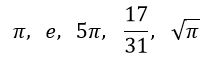2. Show that 3i is an algebraic number by finding a polynomial with rational coefficients that has this number as a solution.

3. Your friend Cameron has claimed that the square root of 5 is a transcendental number, since it is an irrational number.

• Is Cameron correct?
• If not, prove that Cameron is incorrect by finding a polynomial with rational coefficients for which the square root of 5 is a solution.

### Solutions

1. In that list, the algebraic number is 17 / 31. This is an algebraic number because it is a rational number - and every rational number is algebraic. The easiest and quickest polynomial to find that has this number as a solution is: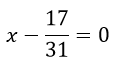We can multiply both sides of the equation by 31 to get a different polynomial equation with integer coefficients for which 17 / 31 is a solution: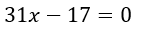2. As 3i is the square root of -9, we know that 3i is a solution to this equation: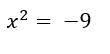Moving the -9 to the other side of the equation, we have a polynomial with rational coefficients, set equal to zero, for which 3i is a solution.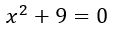3. Cameron is incorrect - all transcendental numbers are irrational, but not all irrational numbers are transcendental. The square root of 5 is a solution to the equation: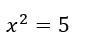Subtracting 5 from both sides of the equation gives a polynomial with rational coefficients, set equal to zero, for which the square root of 5 is a solution: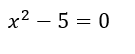### Register to view this lesson

Are you a student or a teacher?